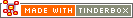^capitalize( data )^Code Type: Export Mark-up Code Scope of Action: n/a

^capitalize( data )^

This text transform "filter" evaluates and transforms the data argument, by capitalizing the first letter of each word.

^capitalize(an example)^ outputs An Example

For note "a sample note"

^capitalize(^get(this,Name^)^ outputs A Sample Note

Characters other than the first in each word aren't aren't touched.

^capitalize(aN exaMple)^ outputs AN ExaMple

In the latter case you may need to lowercase the string first.

 Up: Export Mark-up Previous: ^setRoot( [newRoot] )^ Next: ^cloud( item, count] )^

[Last updated: 14 Dec 2009, using v5.0]